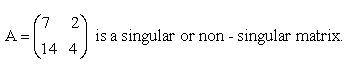Name: ___________________Date:___________________

 Email us to get an instant 20% discount on highly effective K-12 Math & English kwizNET Programs!

### High School Mathematics - 26.8 Singular and non-Singular Matrix

 Singular Matrix:- A square matrix whose determinant is zero is called a singular matrix. Non-Singular Matrix:- A sqare matrix whose determinant is non-zero is called a non-singular matrix. Directions: Check the given matrix is singular matrix or non-singular matrix. Also write at least ten examples for singular and non singular matrix.Name: ___________________Date:___________________

### High School Mathematics - 26.8 Singular and non-Singular Matrix

 Q 1:Non-Singular MatrixSingular Matrix Q 2:Non-Singular MatrixSingular Matrix Question 3: This question is available to subscribers only! Question 4: This question is available to subscribers only!